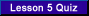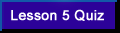VA FileMan V. 22 Key and Index Tutorial[<-- Previous Lesson][Next Lesson -->]

Part 1 - Regular Indexes

 Lesson 5. Whole-File Indexes

In this lesson you will create a whole-file index based on the fields in the EMAIL multiple of the ZZINDIVIDUAL test file. This lesson will also illustrate a use for computed cross-reference values.

Exercise 5.1.  Create a Whole-File Index Based on EMAIL NAME and EMAIL DOMAIN

In this exercise you will create a whole-file index that contains one computed subscript based on the EMAIL NAME and the EMAIL DOMAIN fields within the EMAIL multiple. The subscript in the index will look like:

email_name@email_domain

 Step 1. First, follow the menu path to the option Cross Reference a Field or File as shown below:
 VA FileMan   Utility Functions      Cross Reference a Field or File

 Step 2. Enter N for New at the prompt:
 What type of cross-reference (Traditional or New)? Traditional//N

 Step 3. Select the ZZINDIVIDUAL file and EMAIL subfile. Answer yes when prompted if you want to create a new Index.
MODIFY WHAT FILE: ZZINDIVIDUAL// <Enter>
Select Subfile: EMAIL <Enter> (Subfile #662nnn.02)

There are no INDEX file cross-references defined on subfile #662nnn.02. Want to create a new Index for this file? No// Y <Enter> YES

 Note: The fields you will select as cross-reference values in our index will be fields in the EMAIL subfile.

 Step 4. For type of index, enter: REGULAR.
 Type of index: REGULAR// REGULAR

 Step 5. Because you selected a subfile (the EMAIL multiple), VA FileMan asks you whether you want to index the whole ZINDIVIDUAL file. Press Enter to select the default YES.
Want to index whole file ZZINDIVIDUAL (#662nnn)? Yes// <Enter> YES

 Note: If you answered NO here, the index would be stored at the subfile level. This would allow you to lookup an entry in the EMAIL subfile for a specific record in the ZZINDIVIDUAL file.

 Step 6. When asked how the index should be used, select SORTING ONLY:
```Want index to be used for Lookup & Sorting
or Sorting Only: LOOKUP & SORTING// SORTING ONLY
```
 Note: You want VA FileMan to use this index for lookup only if you explicitly specify the index in the call. If you choose LOOKUP & SORTING here, VA FileMan would automatically use this index whenever you passed the "M" flag to a lookup API.

 Step 7. For Index name select the default AC.
`Index Name: AC// <Enter> AC `
 Note: Sorting Only indexes must have names that start with the letter "A".

 Step 8. You are now presented with the two-page ScreenMan form for editing the properties of your index. On the first page, enter the following as the Short Description:
This is a whole-file index with the computed subscript email name@email domain.

 Number: nnn EDIT AN INDEX Page 1 of 2 File:Index Name: 662nnn AC Root File: 662nnn.02 Root Type: WHOLE FILE Short Description: This is a whole-file index with the computed subscript email name@email domain. Description (wp): (empty) Type: REGULAR Activity:Execution: IRFIELD Use: SORTING ONLY COMMAND:                     Press <>PF1>H for help   Insert
 Note: We're defining a whole-file index (Root type=WHOLE FILE), that contains fields at a subfile level (Root file=662nnn.02), but that resides at the top level (File = 662nnn), the level at which you can use the index to lookup entries.

 Step 9. Press or to go to page 2 of the ScreenMan form.

 Step 10. Create two cross-reference values of type FIELD. Define the first as the EMAIL NAME field (#.01), and the second as the EMAIL DOMAIN field (#1) as shown below:
 ```Number: nnn EDIT AN INDEX Page 2 of 2 ``` ```CROSS-REFERENCE VALUES: Order... Subscr Type Length Field or Computed Expression -------- ------ ---- ------ ---------------------------- 1 1 FIELD 30 EMAIL NAME (#.01) 2 2 FIELD 30 EMAIL DOMAIN (#1) Set Logic: S ^DIZ(662nnn,"AC",\$E(X(1),1,30),\$E(X(2),1,30),DA(1),DA)="" Kill Logic: K ^DIZ(662nnn,"AC",\$E(X(1),1,30),\$E(X(2),1,30),DA(1),DA) Whole Kill: K ^DIZ(662nnn,"AC") Set Condition: Kill Condition:``` COMMAND:                               Press <>PF1>H for help    Insert
 Note: These two fields (EMAIL NAME and EMAIL DOMAIN) will not be used as subscripts in our index. Instead, you will create a single subscript based on those two fields.

 Step 11. Tab to the "Subscr" column in the ScreenMan form, page 2, and delete the values 1 and 2.
 Note: Since you are creating a Regular index, VA FileMan assumes that all fields you add as cross-reference values in the index will be used as subscripts and automatically assigns subscript numbers to them. Most of the time, this assumption is correct. However, if a cross-reference value is not to be used as a subscript in the index, as in this example, you must remember to delete the automatically generated subscript number.As you will see in Lesson 6, when you create a MUMPS cross-reference, FileMan assumes that cross-reference values will not be used as subscripts in an index.

 Step 12. Tab to the next column labeled "Order…" on the third row of the table, type in the number 3 and press Enter.

 Step 13. At the "CROSS-REFERENCE VALUES TYPE OF VALUE:" prompt enter "C" for COMPUTED.
 CROSS-REFERENCE VALUES TYPE OF VALUE: C

 Step 14. Enter 1 as the subscript number.

 Step 15. Tab to "Computed Code," enter:
 `S X=\$\$UP^XLFSTR(X(1)_"@"_X(2))`
 Step 16. Press C to close the pop-up window.

 Step 17. Delete all the values in the "Length" column. Both EMAIL NAME and EMAIL DOMAIN are free text fields from 1 to 20 characters in length. Our computed subscript, EMAIL NAME@EMAIL DOMAIN should therefore never exceed 41 characters (1 to 20 for each field combined and 1 for the "@" sign) in length, well within the limits of the M portability standards.Here is how page 2 of the ScreenMan form should look:
 ```Number: nnn EDIT AN INDEX Page 2 of 2 ``` ```CROSS-REFERENCE VALUES: Order... Subscr Type Length Field or Computed Expression -------- ------ ---- ------ ---------------------------- 1 FIELD EMAIL NAME (#.01) 2 FIELD EMAIL DOMAIN (#1) 3 1 COMPUTED S X=\$\$UP^XLFSTR(X(1)_"@"_X(2)) Set Logic: S ^DIZ(662nnn,"AC",X(3),DA(1),DA)="" Kill Logic: K ^DIZ(662nnn,"AC",X(3),DA(1),DA) Whole Kill: K ^DIZ(662nnn,"AC") Set Condition: Kill Condition: ``` COMMAND:                    Press <>PF1>H for help    Insert

 Step 18. Press E to save the changes and exit the form.

 Step 19. If asked whether you to build the index now, answer Yes:
 ```Do you want to build the index now? YES// ```
 Step 20. From programmer mode, do a %G listing to look at the "AC" index that was built:
 ```>D ^%G Global ^DIZ(662nnn,"AC" DIZ(662nnn,"AC" ^DIZ(662nnn,"AC","BASIL@ABC.DEF.COM",12,1) = ^DIZ(662nnn,"AC","BSNOW@XXX.YYY.COM",12,2) = ^DIZ(662nnn,"AC","CHERVIL@XXX.YYY.COM",11,1) = ^DIZ(662nnn,"AC","CPUDDLES@ABC.DEF.COM",11,2) = ^DIZ(662nnn,"AC","DILL@ABC.DEF.COM",5,1) = ^DIZ(662nnn,"AC","DTIDE@XXX.YYY.COM",5,2) = ^DIZ(662nnn,"AC","GINGER@AAA.BBB.COM",9,1) = ^DIZ(662nnn,"AC","HAZEL.FROST@ABC.DEF.COM",7,1) = ^DIZ(662nnn,"AC","HAZEL@ABC.DEF.COM",7,2) = ^DIZ(662nnn,"AC","HERB@ABC.DEF.COM",3,1) = ^DIZ(662nnn,"AC","HOLLY@ABC.DEF.COM",13,1) = ^DIZ(662nnn,"AC","HOLLYRIVERS@XXX.YYY.COM",13,2) = ^DIZ(662nnn,"AC","HWATERS@ABC.DEF.COM",3,2) = ^DIZ(662nnn,"AC","JASMINE.GEISER@AAA.BBB.COM",2,1) = ^DIZ(662nnn,"AC","JASMINE@XXX.YYY.COM",2,2) = ^DIZ(662nnn,"AC","MARIGOLD@AAA.BBB.COM",4,2) = ^DIZ(662nnn,"AC","MLAKE@XXX.YYY.COM",4,1) = ^DIZ(662nnn,"AC","PERIWINKLE@ABC.DEF.COM",10,1) = ^DIZ(662nnn,"AC","PWELLS@XXX.YYY.COM",10,2) = ^DIZ(662nnn,"AC","RC@AAA.BBB.COM",1,1) = ^DIZ(662nnn,"AC","ROSE@XXX.YYY.COM",1,2) = ^DIZ(662nnn,"AC","SAFFRON@AAA.BBB.COM",8,1) = ^DIZ(662nnn,"AC","SAGEBROOKS@AAA.BBB.COM",6,1) = ^DIZ(662nnn,"AC","SRIPPLE@ABC.DEF.COM",8,2) = Global ^ ```

End of Exercise 5.1.Select this link to test yourself on what you've learned in this lesson.

 [<-- Previous Lesson]   [Intro]     5         [Next Lesson -->]

Reviewed/Updated: March 20, 2007